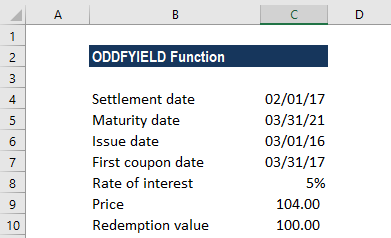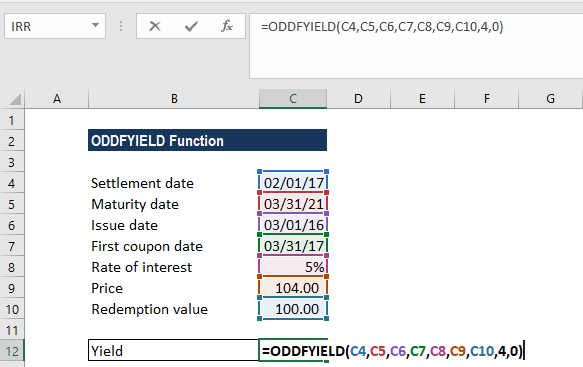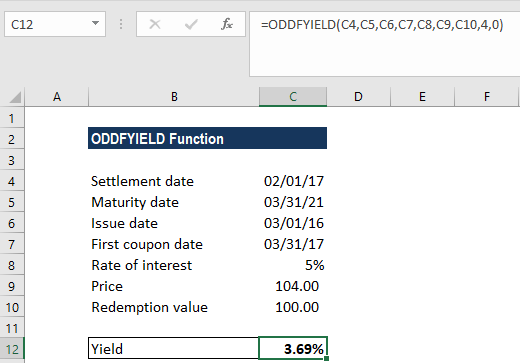ODDFYIELD Function

Calculates the yield of a security with an odd (short or long) first period

What is the ODDFYIELD Function?

The ODDFYIELD Function is categorized under Excel Financial functions. It helps calculate the yield of a security with an odd (short or long) first period.

In financial analysis, some bonds come with irregular first or last periods. Due to the irregular first or last period, the payment doesn’t fit in any of the given patterns. If we wish to calculate the yield of a security, we can use the ODDFYIELD function for such bonds.

Formula

=ODDFYIELD(settlement, maturity, issue, first_coupon, rate, yld, redemption, frequency, [basis])

The ODDFYIELD uses the following arguments:

1. Settlement (required argument) – This is the security’s settlement date. It is the date after the issue date when the security is traded to the buyer.
2. Maturity (required argument) – This is the security’s maturity date. It is the date when the security expires.
3. Issue (required argument) – This is the security’s issue date.
4. First_coupon (required argument) – This is the security’s first coupon date.
5. Rate (required argument) – The security’s interest rate.
6. Yld (required argument) – The security’s annual yield.
7. Redemption (required argument) – This is the security’s redemption value per \$100 face value.
8. Frequency (required argument) – This is the number of coupon payments per year. For annual payments, frequency = 1; for semi-annual, frequency = 2; for quarterly, frequency = 4.
9. Basis (optional argument) – It is the type of day count basis to use. The possible values of the basis are as follows:

BasisDay Count basis
0 or omittedUS(NASD) 30/360
1Actual/actual
2Actual/360
3Actual/365
4European 30/360

How to use the ODDFYIELD Function in Excel?

To understand the uses of the ODDFYIELD function, let’s consider an example:

Example

Let’s assume we need to calculate the yield per \$100 face value of a security with issue date Mar. 1, 2016, settlement date Feb. 1, 2017, first coupon date Mar. 31, 2017 and the maturity date Mar. 31, 2021. The rate of interest is 5%, the price is \$104 and the redemption value is \$100. Payments are made quarterly and the US (NASD) 30/360 day count basis is used:The formula used is:We get the result below:The above function returned the value of 3.69%. In the example:

• The date arguments were supplied to the ODDFYIELD function as references to cells containing dates.
• The rate argument was entered as a percentage (5%). However, we can enter the arguments as the simple numeric value 0.005.
• As we intend to omit the [basis] argument, the function uses the default value 0 (denoting the US (NADS) 30/360 day count basis).

Notes about the ODDFYIELD Function:

1. #NUM! error – Occurs when:
• The given issue date is greater than or equal to the settlement date.
• The given settlement date is greater than or equal to the first_coupon date.
• The first_coupon date given is greater than or equal to the maturity date.
• We provided invalid numbers for the rate, pr, redemption, frequency or [basis] arguments. That is if either rate is less than 0; pr is less than 0; redemption is less than or equal to 0; frequency is any number other than 1, 2, or 4; or [basis] is any number other than 0, 1, 2, 3, or 4).
2. #VALUE! error – Occurs when:
• The given settlement, maturity, issue or first coupon arguments are not valid Excel dates.
3. Any of the given arguments is non-numeric.

Thanks for reading CFI’s guide to the Excel ODDFYIELD function. By taking the time to learn and master these Excel functions, you’ll significantly speed up your financial analysis. To learn more, check out these additional CFI resources:

• Excel Functions for Finance
• Advanced Excel Formulas Course
• Advanced Excel Formulas You Must Know
• Excel Shortcuts for PC and Mac

Free Excel Tutorial

To master the art of Excel, check out CFI’s FREE Excel Crash Course, which teaches you how to become an Excel power user.  Learn the most important formulas, functions, and shortcuts to become confident in your financial analysis.

Launch CFI’s Free Excel Course now to take your career to the next level and move up the ladder!